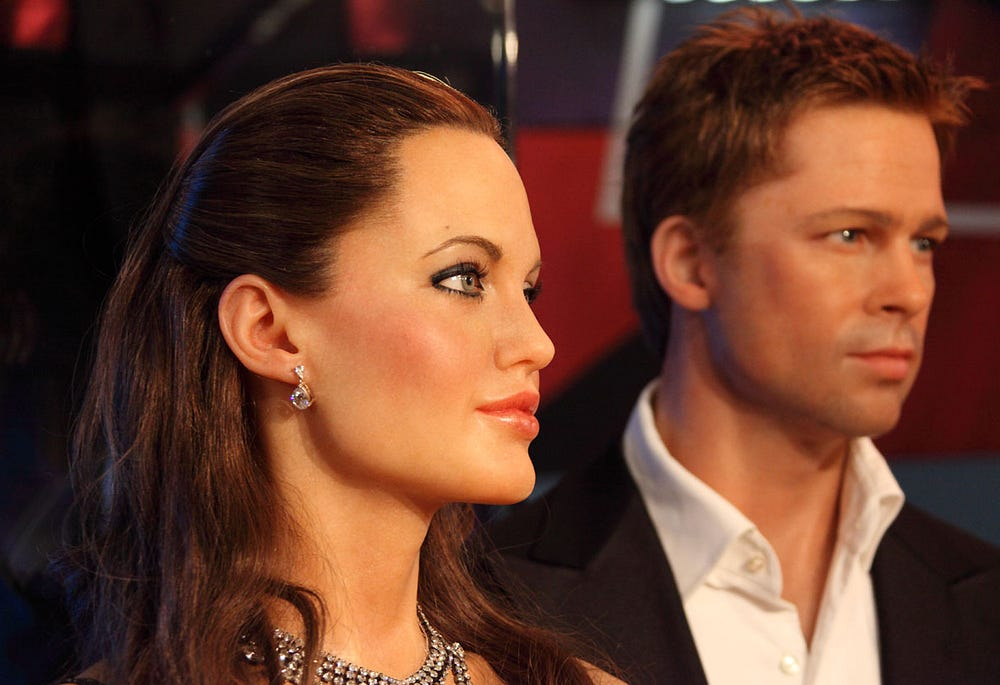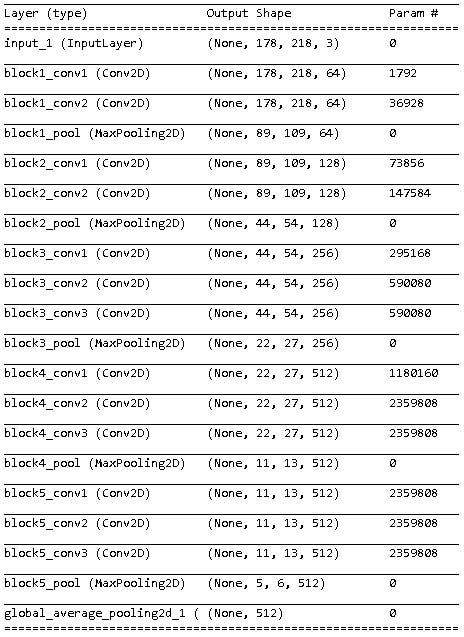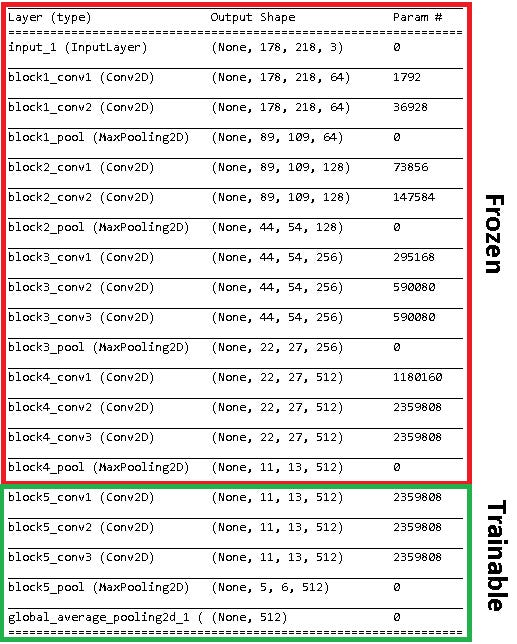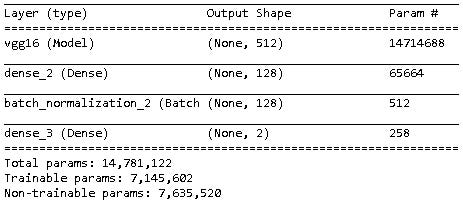# Gender Identification with Deep Learning

Source: Deep Learning on Medium

Fine-tuning pretrained convolutional neural networks on celebritiesSource: Public Domain Pictures

### Summary

I wanted to build a model to identify gender from images of human faces. By fine-tuning the pretrained convolutional neural network VGG16, and training it on images of celebrities, I was able to obtain over 98% accuracy on the test set. The exercise demonstrates the utility of engineering the architecture of pretrained models to complement the characteristics of the dataset.

### Task

Typically, a human can distinguish a man and a woman in the photo above with ease, but it’s hard to describe exactly why we can make that decision. Without defined features, this distinction becomes very difficult for traditional machine learning approaches. Additionally, features that are relevant to the task are not expressed in the exact same way every time, every person looks a little different. Deep learning algorithms offer a way to process information without predefined features, and make accurate predictions despite variation in how features are expressed. In this article, we’ll apply a convolutional neural network to images of celebrities with the purpose of predicting gender. (Disclaimer: the author understands appearance does not have a causative relationship with gender)

### Tool

Convolution neural networks (ConvNets) offer a means to make predictions from raw images. A hallmark of the algorithm is the ability to reduce the dimensionality of images by using sequences of filters that identify distinguishing features. Additional layers in the model help us emphasize the strength of often nonlinear relationships between the features identified by the filters and the label assigned to the image. We can adjust weights associated with the filters and additional layers to minimize the error between the predicted and observed classifications. Sumit Saha offers a great explanation that is more in-depth: https://towardsdatascience.com/a-comprehensive-guide-to-convolutional-neural-networks-the-eli5-way-3bd2b1164a53

There are a number of pretrained ConvNets that have been trained to classify a range of images of anything from planes to corgis. We can save computation time and overcome some sampling inadequacy by employing the weights of pretrained models and fine-tuning them for our purpose.

### Dataset

The CelebA dataset contains over 200K images of celebrities labeled with 20 attributes including gender. The images are from the shoulders up, so most of the information is in the facial features and hair style.Example image available from CelebA

### Modeling

#### Feature Extraction

We’re going to use the VGG16 pretrained model and fine tune it to best identify gender from the celebrity images.

`vgg=VGG16(include_top=False, pooling=’avg’, weights=’imagenet’,input_shape=(178, 218, 3))`

We use “include_top=False” to remove the fully connected layer designed for identifying a range of objects the VGG16 was trained to identify (e.g. apples, corgis, scissors), and we download the weights associated with the ImageNet competition.Table 1: Architecture for the VGG16 model

Table 1 shows the convolutional architecture for VGG16; there are millions of weights for all the convolutions that we can choose to either train or keep frozen at the pretrained values. By freezing all the weights of the model, we risk underfitting it because the pretrained weights were not specifically estimated for our particular task. In contrast, by training all the weights we risk overfitting the model without very large amounts of data because the model will begin “memorizing” the training images given the flexibility of the high parameterization. We’ll attempt a compromise by training the last convolutional block:

`# Freeze the layers except the last 5for layer in vgg.layers[:-5]: layer.trainable = False`
`# Check the trainable status of the individual layersfor layer in vgg.layers: print(layer, layer.trainable)`Table 2: Architecture of VGG16 model after turning final layers on

The first convolutional blocks in the VGG16 models are identifying more general features like lines or blobs, so we want to keep the associated weights. The final blocks identify more fine scale features (e.g. angles associated with the wing tip of an airplane), so we’ll train those weights given our images of celebrities.

#### Model Compilation

Following feature extraction by the convolutions, we’ll add two dense layers to the model that enable us to make predictions about the image given the features identified. You could use a single dense layer, but an additional hidden layer allows predictions to be made given a more sophisticated interpretation of the features. Too many dense layers may cause overfitting.

`# Create the modelmodel = models.Sequential()`
`# Add the VGG16 convolutional base modelmodel.add(vgg)# Add new layersmodel.add(layers.Dense(128, activation=’relu’))model.add(layers.BatchNormalization())model.add(layers.Dense(2, activation=’sigmoid’))`

We added a batch normalization layer that will scale our hidden layer activation values in a way to reduce overfitting and computation time. The last dense layer makes predictions about gender (Table 3).Table 3: Custom Model Architecture

Because we are allowing the model to train convolutional layers and dense layers, we’ll be estimating millions of weights (Table 3). Given the depth of the network we built, picking the best constant learning rate for an optimizer like stochastic gradient decent would be tricky; instead we’ll use the ADAM optimizer, that adjusts the learning rate to make smaller steps further into training.

`model.compile(optimizer=’adam’, loss=’binary_crossentropy’, metrics=[‘accuracy’])`

Before we start training the model, I’ll briefly note two useful functions in Keras, EarlyStopping and ModelCheckpoint. EarlyStopping allows us to stop the training process when the validation loss doesn’t improve given a certain number of epochs. ModelCheckpoint allows us to then save that model

`from tensorflow.python.keras.callbacks import EarlyStopping, ModelCheckpoint`
`# Stop training when validation gets worstes = EarlyStopping(monitor=’val_loss’, mode=’min’, verbose=1, patience=2)`
`# save best modelmc = ModelCheckpoint('C:/Users/w10007346/Dropbox/CNN/Gender ID/best_model_2.h5', monitor='val_loss', mode='min', verbose=1, save_best_only=True)`
`# store both callbacks cb_list = [es,mc]`

Using Keras, we’ll set up our data generators to feed our model, and fit the network to our training set, employing the EarlyStopping and ModelCheckpoint functions as callbacks during the .fit command.

`data_generator = ImageDataGenerator(preprocessing_function=preprocess_input)`
`train_generator = data_generator.flow_from_directory( ‘C:/Users/w10007346/Pictures/Celeb_sets/train’, target_size=(178, 218), batch_size=12, class_mode=’categorical’)`
`validation_generator = data_generator.flow_from_directory( ‘C:/Users/w10007346/Pictures/Celeb_sets/valid’, target_size=(178, 218), batch_size=12, class_mode=’categorical’)`
`model.fit_generator( train_generator, epochs=20, steps_per_epoch=2667, validation_data=validation_generator, validation_steps=667, callbacks=cb_list)`

After 6 epochs, the model achieved a maximum validation accuracy of 98%. Now to apply to the test set.

#### Testing

We have a test set of 500 images per gender. The model will give us predicted probabilities for each image fed through the network and we can simply take the maximum value of those probabilities as the predicted gender.

`# generate data for test set of imagestest_generator = data_generator.flow_from_directory( ‘C:/Users/w10007346/Pictures/Celeb_sets/test’, target_size=(178, 218), batch_size=1, class_mode=’categorical’, shuffle=False)`
`# obtain predicted activation values for the last dense layertest_generator.reset()pred=saved_model.predict_generator(test_generator, verbose=1, steps=1000)`
`# determine the maximum activation value for each samplepredicted_class_indices=np.argmax(pred,axis=1)`
`# label each predicted value to correct genderlabels = (test_generator.class_indices)labels = dict((v,k) for k,v in labels.items())predictions = [labels[k] for k in predicted_class_indices]`
`# format file names to simply male or femalefilenames=test_generator.filenamesfilenz=for i in range(0,len(filenames)): filenz.append(filenames[i].split(‘\\’))filenz=filenz[1:]`
`# determine the test set accuracymatch=[]for i in range(0,len(filenames)): match.append(filenz[i]==predictions[i])match.count(True)/1000`

Our model predicted the gender of celebrities with 98.2% accuracy! That’s pretty comparable to human capabilities.

Does the model generalize to non-celebrities? Lets try on the author. The model did well with a recent picture of the author.

The predicted probability for this image was 99.8% male.

The model also did well with the author’s younger, mop-head past; it predicted 98.6% male.

### Conclusion

This exercise demonstrates the power of fine-tuning pretrained ConvNets. Each application will require a different approach to optimize the modeling process. Specifically, the architecture of the model needs to be engineered in a way that complements the characteristics of the dataset. Pedro Marcelino offers a great explanation of general rules for adapting the fine-tuning process to any dataset: https://towardsdatascience.com/transfer-learning-from-pre-trained-models-f2393f124751

I appreciate any feedback and constructive criticism on this exercise. The code associated with the analysis can be found on github.com/njermain